### GATE 1996 ECE Video Solutions on Digital Circuits (Digital Electronics)

1. Schottky clamping is resorted in TTL gates
a. To reduce propagation delay
b. To increase noise margins
c. To increase packing density
d. To increase fan-out

2. A pulse train can be delayed by a finite number of clock periods using a
a. Serial In Serial Out shift register
b. Serial In Parallel Out shift register
c. Parallel In serial Out shift register
d. Parallel In parallel Out shift register
Solution :

3. A 12 bit ADC is operating with a 1 µsec clock period and the total conversion time is seen to be 14 µsec. the ADC must be of the
a. Flash type
b. Counting type
c. Integrating type
d. Successive Approximation type

4. The total number of memory accesses involved (inclusive of the op-code fetch), when an 8085 processor executes the instruction LDA 2003 is
a. 1
b. 2
c. 3
d. 4
Solution :

5. A Dynamic RAM cell which holds 5 volts has to be refreshed every 20 ms, so that the stored voltage does not fall by more than 0.5 volts. If the cell has a constant discharge current of 0.1 pA, the storage capacitance of the cell is

6. A 10 bit ADC with full scale output voltage of 10.24 volts is designed to have a ±LSB/2 accuracy. If the ADC is calibrated at 25oC and the operating temperature ranges from 0oC to 50oC, then the maximum net temperature coefficient of the ADC should not exceed
a. ± 200 µV/oC
b. ± 400 µV/oC
c. ± 600 µV/oC
d. ± 800 µV/oC
Solution :

7. A memory system of size 26K bytes is required to be designed using memory chips which have 12 address lines and 4 data lines each. The number of such chips required to design the memory system is
a. 2
b. 4
c. 8
d. 13

8. The following sequence of instructions are executed by an 8085 microprocessor:
1000 LXI SP, 27FFH
1003 CALL 1006
1006 POP H
The contents of the stack pointer (SP) and the HL register pair on completion of execution of these instructions are
a. SP = 27FF, HL = 1003
b. SP = 27FD, HL = 1003
c. SP = 27FF, HL = 1006
d. SP = 27FD, HL = 1006
Solution :

9. Given the Boolean function F in three variables R, S and T as
F = R’ST’ + RS’T + RST
a. Express F in the minimum sum of products form
b. Express F in the minimum product of sums form
c. Assuming that both true and complement forms of the input variables are available, draw a circuit to implement F using the minimum number of 2 input NAND gates only.

10. A state machine is required to cycle through the following sequence of states:
ABC : 000 -> 010 -> 111 -> 100 -> 011 -> 101.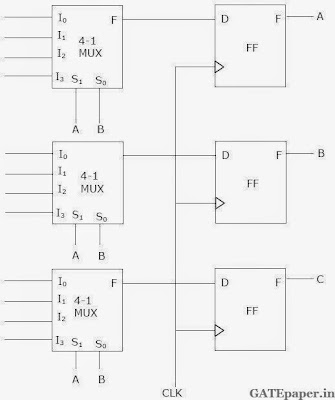One possible implementation of the state machine is shown in figure. Specify what signals should be applied to each of the multiplexer inputs.

11. A 4 bit shift register, which shifts 1 bit to the right at every clock pulse, is initialized to values 1000 for (Q0Q1Q2Q3). The D input is derived from Q0, Q2 and Q3 through two XOR gates as shown in figure.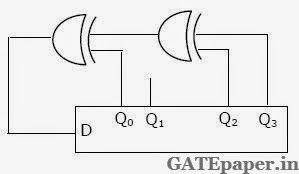a. Write the 4 bit values (Q0Q1Q2Q3) after each clock pulse till the pattern (1000) reappears on (Q0Q1Q2Q3).
b. To what values should the shift register be initialized so that the pattern (1001) occurs after the first clock pulse?

12. It is desired to generate the following three Boolean functions using an OR array as shown in figure, where P1 to P5 are the product terms in one or more of the variables a, a’, b, b’, c and c’.Write down the terms P1, P2, P3, P4 and P5.

### GATE 1997 ECE Video Solution on Digital Circuits (Digital Electronics)

1. Each cell of a Static Random Access Memory contains
a. 6 MOS transistors
b. 4 MOS transistors and 2 capacitors
c. 2 MOS transistors and 4 capacitors
d. 1 MOS transistor and 1 capacitor

2. A two bit binary multiplier can be implemented using
a. 2 input AND gates only
b. 2 input XOR gates and 2 input AND gates only
c. Two 2 input NOR gates and one XOR gate
d. XOR gates and shift registers

3. In standard TTL, the ‘Totem pole’ stage refers to
a. Multi emitter input stage
b. Phase splitter
c. Output buffer
d. Open collector output stage

4. The inverter 74ALS04 has the following specifications:
IOHmax = -0.4 mA
IOLmax = 8 mA
IIHmax = 20 µA
IILmax = -0.1 mA
The fan out based on the above will be
a. 10
b. 20
c. 60
d. 100

5. The output of the logic gate shown is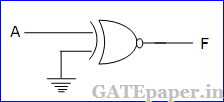a. 0
b. 1
c. A
d. A’

6. In 8085 µP system, the RST instruction will cause an interrupt
a. Only if an interrupt service routine is not being executed
b. Only if a bit in the interrupt mask is made 0
c. Only if interrupts have been enabled by an EI instruction
d. None of the above
Solution :

7. The decoding circuit is shown in figure, has been used to generated the active low chip select signal for a microprocessor peripheral (The address lines are designated as A0 to A7 for I/O addresses).a. 60H – 63H
b. A4 – A7H
c. 30 – 33H
d. 70 – 73H

8. In a JK flip flop, we have J = Q’ and K = 1. Assume the flip flop was initially cleared and then clocked for 6 pulses, the sequence at the Q output will be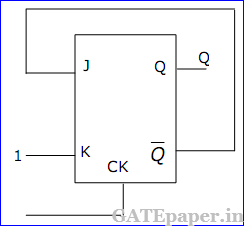a. 010000
b. 011001
c. 010010
d. 010101

9. For the NMOS logic gate shown, the logic function implemented is10. The Boolean function A + BC is a reduced form of
a. AB + BC
b. (A + B)(A + C)
c. A’B + AB’C
d. (A + C)B

11. The following instructions have been executed by an 8085 µP
For which address will the next instruction be fetched?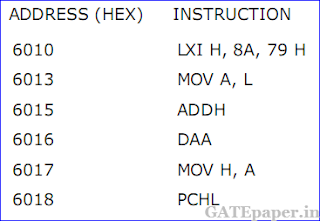a. 6019
b. 6379
c. 6979
d. None of the above
Solution :

12. A signed integer has been stored in a byte using the 2’s complement format. We wish to store the same integer in a 16 bit word. We should
a. copy the original byte to the less significant byte of the word and fill the more significant with zeros
b. copy the original byte to the more significant byte of the word and fill the less significant with zeros
c. copy the original byte to the less significant byte of the word and make fit of the more significant byte equal to the most significant bit of the original byte
d.copy the original byte to the less significant byte of the word as well as the more significant byte of the word

13. Match the following :14. Match the following, while moving data between registers of the 8085 and the stack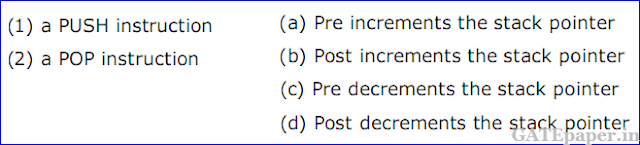Solution :

15. Circuit shown in the figure is an NMOS shift register. All transistors are NMOS enhancement type with threshold voltage VT = 1 volt. Supply used is VDD = 5 volts.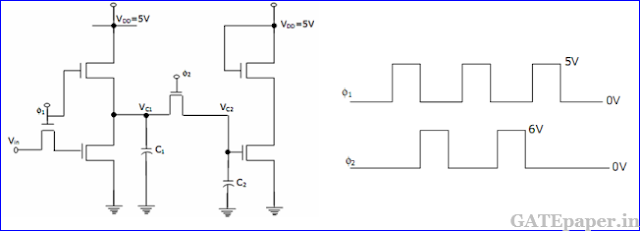Two non- overlapping clocks ø1 and ø2 are as shown in the figure is and have large pulse widths.
All capacitors are initially discharged and the Vin = 0 volts is applied. If values of capacitors are C1 = 2 pF and C2 = 1 pF. Find out voltage VC2 on capacitor C2 after ø2 goes low. Neglect body effect on VT in your evaluation.

16. A sequence generator is shown in figure. The counter status (Q0Q1Q2) is initialized to 010 using preset/clear inputs.The clock has a period of 50 ns and transitions take place at the rising clock edge.
a. Give the sequence generates a tQ0 till repeats.
b. What is the repetition rate of the generated sequence?

17. An 8085 µP uses a 2 MHz crystal. Find the time taken by it to execute the following delay subroutine, inclusive of the call instruction in the calling program.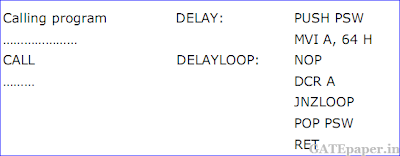You are given that a CALL instruction takes 18 cycles of the system clock, PUSH requires 12 cycles and conditional jump takes 10 cycles if the jump is taken and 7 cycles if it is not. All other instructions used above take (3n + 1) clock cycles, where n is the number of accesses to the memory, inclusive of the opcode fetch.

Solution :

### GATE 1998 ECE Video Solution on Digital Circuits (Digital Electronics)

1. The minimum number of 2 input NAND gates required to implement the Boolean function Z = AB’C, assuming that A, B and C are available, is
a. Two
b. Three
c. Five
d. Six

2. The noise margin of a TTL gate is about
a. 0.2 volts
b. 0.4 volts
c. 0.6 volts
d. 0.8 volts

3. In the figure shown is A = 1 and B = 1, the input B is now replaced with a sequence 101010....., the output X and Y will bea. Fixed at 0 and 1 respectively
b. X = 1010... while Y = 0101....
c. X = 1010.... and Y = 1010....
d. Fixed at 1 and 0 respectively

4. An equivalent 2’s complement representation of the 2’s complement number is 1101 is
a. 110100
b. 001101
c. 110111
d. 111101

5. An I/O processor control the flow of information between
a. Cache memory and I/O devices
b. Main memory and I/O devices
c. Two I/O devices
d. Cache and main memory

6. Two 2’s complement numbers having sign bits x and y are added and the sign bit of the result is z. Then, the occurrence of overflow is indicated by the Boolean function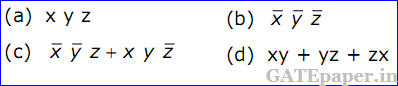7. The advantage of using a dual slope ADC in a digital voltmeter is that
a. Its conversion time is small
b. Its accuracy is high
c. It gives output in BCD format
d. It does not require a comparator

8. For the identity, AB + A’C + BC = AB + A’C, the dual form is9. An instruction used to set the carry flag in a computer can be classified as
a. Data transfer
b. Arithmetic
c. Logical
d. Program control

10. The figure is shows a mod – K counter, here K is equal to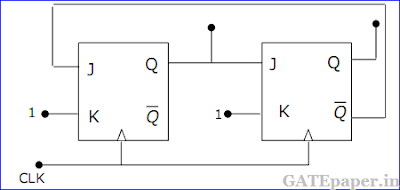a. 1
b. 2
c. 3
d. 4

11. The current I flowing through resistance ‘r’ in the circuit shown is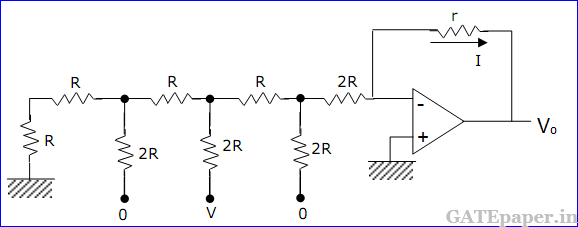a. -V/12R
b. V/12R
c. V/6R
d. V/3R

12. The K – map for a Boolean function is shown in the figure. The number of essential prime implicants for this function isa. 4
b. 5
c. 6
d. 7

13. The mod – 5 counter is shown in the figure counts through states Q2Q1Q0 = 000, 001, 010, 011 and 100.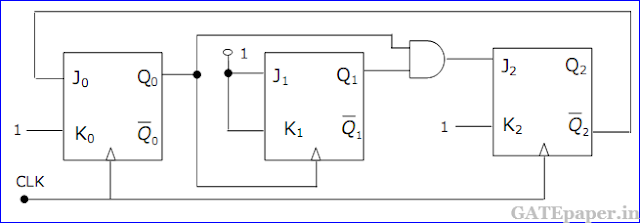a. Will the counter lockout if it happen to be in any one of the unused states?
b. Find the maximum rate at which the counter will operate satisfactorily. Assume the propagation delays of flip-flop and AND gate to be tFF and tA respectively.

14. For the TTL circuit shown in the figure, find the current flowing through the collector of transistor Q4, when Vo = 0.2 volts. Assume VCEsat = 0.2 volts, β = 100 and VBEsat = 0.7 volts. The α of Q1 in its inverse active mode is 0.01.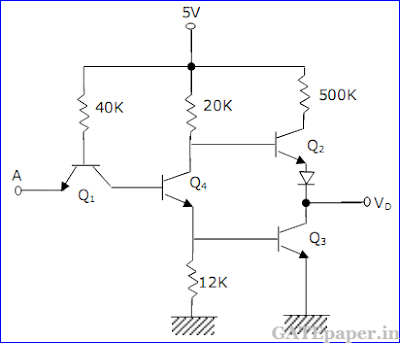15. Write a short assembly language program, without using any arithmetic instruction, to store hexadecimal 5D in the flag register of 8085 microprocessor. Data in other registers of the processor must not alter upon executing this program.

Solution :

### GATE 1999 ECE Video Solution on Digital Circuits (Digital Electronics)

1. The logical expression Y = A + A’B is equivalent to
a. AB
b. A’B
c. A’ + B
d. A + B

2. A Darlington emitter follower circuit is sometimes used in the output stage of a TTL gate, in order to
a. Increase its IOL
b. Reduce its IOH
c. Increase its speed of operation
d. Reduce power dissipation

3. Commercially available ECL gates uses two ground lines and one negative power supply in order to
a. Reduce power dissipation
b. Increase fan-out
d. Eliminate the effect of power line glitches or the biasing circuit

4. The resolution of a 4 bit counting ADC is 0.5 volts. For an analog input of 6.6 volts, the digital output of the ADC will be
a. 1011
b. 1101
c. 1100
d. 1110

5. The minimized form of the logical expression6. For a binary half – subtractor having two inputs A and B, the correct set of logical expressions for the outputs D (= A minus B) and X (= borrow) are7. The ripple counter shown in the given figure is works as aa. Mod – 3 up counter
b. Mod – 5 up counter
c. Mod – 3 down counter
d. Mod – 5 down counter

8. If CS = A15’A14A13 is used as the chip select logic of a 4K RAM in an 8085 system, then its memory range will be
a. 3000 – 3FFFFH
b. 7000 – 7FFFH
c. 5000 – 5FFFH and 6000 – 6FFFH
d. 6000 – 6FFFH and 7000 – 7FFFH

9. The circuit diagram of a synchronous counter is shown in the given figure. Determine the sequence of states of the counter assuming that the initial state is ‘000’. Give your answer in a tabular form showing the present state QA, QB, QC, J-K inputs (JA, KA, JB, KB, JC, KC) and the next state QA+, QB+, QC+. From the table, determine the modulus of the counter.10. In a certain application, four inputs A, B, C, D (both true and complement forms available) are fed to logic circuit, producing an output F which operates a relay. The relay turns on when F(ABCD) = 1 for the following states of the inputs (ABCD): ‘0000’, ‘0010’, ‘0101’, ‘0110’, ‘1101’ and ‘1110’. States ‘1000’ and ‘1001’ do not occur, and for the remaining states, the relay is off. Minimize F with the help of a Karnaugh map and realize it using a minimum number of 3 input NAND gates.

11. An 8085 assembly language program is given below.Solution :

### GATE 2000 ECE Video Solution on Digital Circuits (Digital Electronics)

1. The number of comparators in a 4 bit flash ADC is
a. 4
b. 5
c. 15
d. 16

2. For the logic circuit shown in the figure, the required input combination (A,B,C) to make the output X = 1 is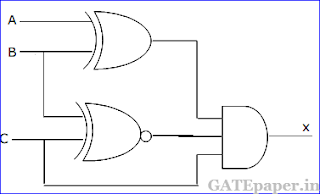a. 1, 0, 1
b. 0, 0, 1
c. 1, 1, 1
d. 0, 1, 1

3. In the 8085 microprocessor, the RST 6 instruction transfers the program execution to the following location
a. 30H
b. 24H
c. 48H
d. 60H

4. An 8 bit successive approximation analog to digital converter has full scale reading of 2.55 volts and its conversion time for an anlog input of 1 volt is 20 µs. The conversion time for a 2 volts input is
a. 10 µs
b. 20 µs
c. 40 µs
d. 50 µs

5. The number of hardware interrupts (which require an external signal to interrupt) present in an 8085 microprocessor are
a. 1
b. 4
c. 5
d. 13

6. The most commonly used amplifier in sample and hold circuits is
a. A unity gain inverting amplifier
b. A unity gain non inverting amplifier
c. An inverting amplifier with a gain of 10
d. An inverting amplifier with a gain of 100

7. For the logic circuit shown, the simplified Boolean expression for the output Y isa. A + B + C
b. A
c. B
d. C

8. For the 4 bit DAC shown, the output voltage Vo isa. 10 volts
b. 5 volts
c. 4 volts
d. 8 volts

9. A sequential circuit using D flip flop and logic gates is shown in figure, where X and Y are the inputs and Z is output. The circuit isa. S-R flip flop with inputs X = R and Y = S
b. S-R flip flop with inputs X = S and Y = R
c. J-K flip flop with inputs X = J and Y = K
d. J-K flip flop with inputs X = K and Y = J

10. The contents of register (B) and Accumulator (A) of 8085 microprocessor are 49H and 3AH respectively. The contents of A and the status of carry flag (CY) and sign flag (S) after executing SUB B instruction are
a. A = F1 , CY = 1 , S = 1
b. A = 0F, CY = 1, S = 1
c. A = F0, CY = 0, S = 0
d. A = 1F, CY = 1, S = 1

11. In the given figure, the J and K inputs of all the four flip-flops are made high. The frequency of the signal at output Y is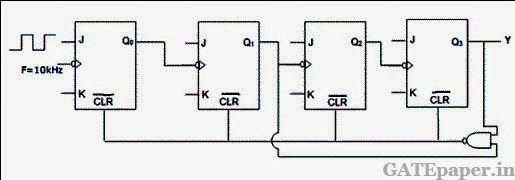a. 0.833 KHz
b. 1.0 KHz
c. 0.91 KHz
d. 0.77 KHz

12. For the CMOS monostable multivibrator of given figure, R = 50 KΩ and C = 0.01 µF, VDD = 5 volts, and the CMOS NOR gates have a threshold voltage (VT) of 1.5 volts. Vin is a trigger pulse (τp << RC) as shown in the figure.a. Plot VA1 and Vo1 as functions of time.
b. Write the equation for VR(t), for t > 0
c. Find the time period of the output pulse.

13. The operating conditions (ON = 1 and OFF = 0) of three pumps (x, y, z) are to be monitored, x = 1 implies that pump x is on. It is required that the indicator (LED) on the panel should glow when a majority of the pumps fail.a. Enter the logical values in the K map in the format shown in figure. Derive the minimal Boolean sum of products expression whose output is zero when a majority of the pumps fail.
b. The above expression is implemented using logic gates, and point P is the output of the circuit, as shown in given figure, P is at 0 volts when a majority of the pumps fails and is at 5 volts otherwise. Design a circuit to derive the LED using this output.
The current through the LED should be 10 mA and the voltage drop across it is 1 volt. Assume that P can source or sink 10 mA and a 5 volts supply is available.

14. A one bit full adder is to be implemented using 8 to 1 multiplexers (MUX).
a. Write the truth table for sum (S) and carry to the next stage (CN), in terms of the two bits (A, B) and the carry from the previous stage (CP). The truth table should be in the ascending order of (A, B, CP) i.e. (000, 001, 010..... etc).
b. Implement S and CN using 8 to 1 multiplexers.

15. The program and machine code for an 8085 microprocessor are given by
The starting address of the above program is 7FFFH. What would happen if it is executed from 8000H?Solution :

### GATE 2001 ECE Video Solution on Digital Circuits (Digital Electronics)

1. The 2’s complement representation of – 17 is
a. 01110
b. 101111
c. 11110
d. 10001

2. For the ring oscillator shown in teh figure, the propagation delay of each inverter is 100 pico sec. What is the fundamental frequency of the oscillator output?a. 10 MHz
b. 100 MHz
c. 1 GHz
d. 2 GHz

3. An 8085 microprocessor based system uses a 4K x8 bit RAM whose starting address is AA00H. The address of the last byte in this RAM is
a. 0FFFH
b. 1000H
c. B9FFH
d. BA00H

4. In the figure, the LEDa. Emits light when both S1 and S2 are closed.
b. Emits light when both S1 and S2 are open.
c. Emits light when only of S1 or S2 is closed.
d. Does not emit light, irrespective of the switch positions.

5. In the TTL circuit in the figure, S2 to S0 are select lines and X7 to X0 are input lines. S0 and X0 are LSBs. The output Y is6. The digital block in the figure, realized using two positive edge triggered D flip-flops. Assume that for t<t0, Q1 = Q2 =0. The circuit in the digital block is given by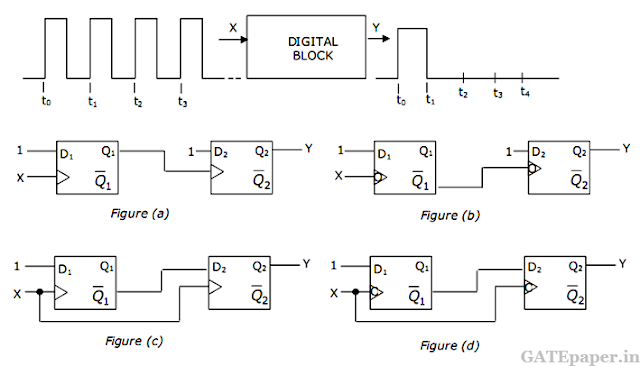7. In the DRAM cell in the figure is the VT of the N-MOSFET is 1 volt. For the following three combinations of WL and BL voltages.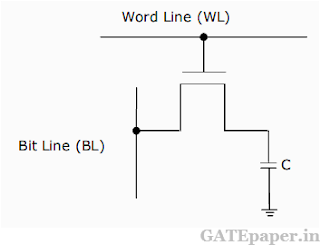a. 5V; 3V; 7V
b. 4V; 3V; 4V
c. 5V; 5V; 5V
d. 4V; 4V; 4V

8. A monochrome video signal that ranges from 0 to 8 volts is digitized using an 8-bit ADC.
a. Determine the resolution of the ADC in V/bit.
b. Calculate the mean squared quantization error.
c. Suppose the ADC is counter controlled. The counter is up counter and positive edge triggered with clock frequency 1 MHz. What is the time taken in seconds to get a digital equivalent of 1.59 volts?

9. In the figure, the output of the oscillator, V1 has 10 volts peak amplitude with zero DC value. The transfer characteristic of the Schmitt inverter is also shown in the figure. Assume that the JK flip-flop is reset at time t=0.a. What is the period and duty cycle of the waveform V2?
b. What is the period and duty cycle of the waveform V3?
c. Sketch V1, V2 and V3 for the duration 0 ≤ t ≤ 6 µsec. Clearly indicate the exact timings when the waveforms V2 and V3 make high to low and low to high transitions.

10. For the digital block shown in the figure, the output Y = f(S3,S2,S2,S0), where S3 is MSB and S0 is LSB. Y is given in terms of minterms as Y = Σm(1,5,6,7,11,12,13,15) and its complement is given as Σm(0,2,3,4,8,9,10,14).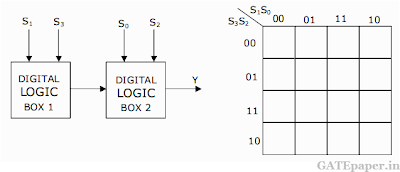a. Enter the logical values in the given Karnaugh map in the figure, for the output Y.
b. Write down the expression for Y in sum of products form using minimum number of terms.
c. Draw the circuit for the digital logic boxes using four 2 input NAND gates only for each of the boxes.

11. Consider the following sequence of instructions for an 8085 microprocessor based system.a. If the program execution begins at the location FF00H, write down the sequence of instructions which are actually executed till a HLT instruction. Assume all Flags are initially RESET.
b. Which of the three ports (PORT1, PORT2 and PORT3) will be loaded with data, and what is the bit pattern of the data?

Solution :

### GATE 2002 ECE Video Solution on Digital Circuits (Digital Electronics)

1. 4 – bit 2’s complement representation of a decimal number is 1000. The number is
a. +8
b. 0
c. -7
d. -8

2. If the input to the digital circuit of the figure, consisting of a cascade of 20 XOR gates is X, then the output Y is equal to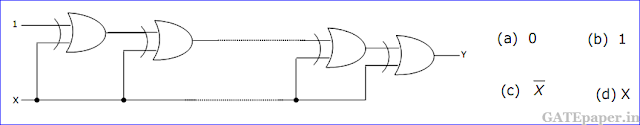3. The number of comparators required in a 3 bit comparator type ADC is
a. 2
b. 3
c. 7
d. 8

4. The gates G1 and G2 in the figure is have propagation delays of 10 ns and 20 ns respectively. If the input Vi makes an abrupt change from logic 0 to 1 at time t = to, then the output waveform Vo is5. The circuit in the figure is ahs two CMOS NOR gates. This circuit functions as a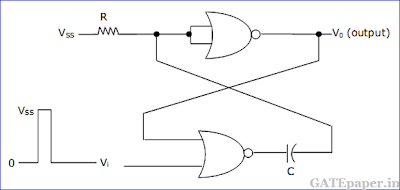a. Flip-flop
b. Schmitt trigger
c. Monostable multivibrator
d. Astable multivibrator

6. If the inputs X3, X2, X1, X0 to the ROM in the figure are 8-4-2-1 BCD numbers, then the outputs Y3Y2Y1Y0 area. Gary code numbers
b. 2-4-2-1 BCD numbers
c. Excess-3 code numbers
d. None of the above

7. Consider the following assembly language program.
MVI B, 87H
MOV A, B
START: JMP NEXT
MVI B, 00H
XRA B
OUT PORT1
HLT
NEXT: XRA B
JP START
OUT PORT2
HLT
The execution of the above program in an 8085 microprocessor will result in
a. An output of 87H at PORT1
b. An output of 87H at PORT2
c. Infinite looping of the program execution with accumulator data remaining at 00H
d. Infinite looping of the program execution with accumulator data alternating between 00H and 87H

8. The inputs to a digital circuit shown in figure is are the external signals A, B and C. Assume complements of A, B and C are not available. The +5 volts power supply (logic 1) and the ground (logic 0) are also available.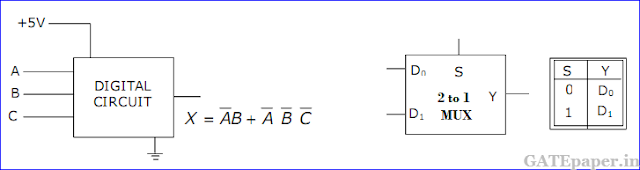a. Write down the output function in its canonical SOP and POS forms.
b. Implement the circuit using only 2 to 1 multiplexers shown in the figure, where S is the data select line, D0 and D1 are the input data lines and Y is the output line. The function table is also given for the multiplexer.

9. It is required to design a binary mod-5 synchronous counter using AB flip-flop, such that the output Q2Q1Q0 changes as 000 -> 001 -> 010..... and so on. The truth table for the AB flip-flop is given in figure.a. Write down the state table for the mod-5 counter
b. Obtain simplified SOP expressions for the inputs A2, B2, A1, B1, A0 and B0 in terms of Q2, Q1, Q0 and their complements.
c. Hence complete the circuit diagram for the mod-5 counter given in the figure using minimum number of 2 input NAND gate only.

10. An 8085 microprocessor operating at 5 MHz clock frequency executes the following routine.
START: MOV A, B
OUT 55H
DCR B
STA FFF8H
JNP START
a. Determine the total number of machine cycles required to execute the routine till the JMP instruction is executed for the first time.
b. Determine the time interval between two consecutive (MEMW)’ signals.
c. If the external logic controls the READSY line so that three WAIT states are introduced in the I/O WRITE machine cycle, determine the time interval between two consecutive (MEMW)’ signals.

Solution :

### GATE 2003 ECE Video Solution on Digital Circuits (Digital Electronics)

1. The number of distinct Boolean expressions of 4 variables is
a. 16
b. 256
c. 1024
d. 65536

2. The minimum number of comparators required to build an 8 bit flash ADC is
a. 8
b. 63
c. 255
d. 256

3. The output of the 74 series of TTL gates is taken from a BJT in
a. Totem pole and Common Collector configuration
b. Either Totem pole or Open Collector configuration
c. Common Base configuration
d. Common Collector configuration

4. Without any additional circuitry, an 8:1 MUX can be used to obtain
a. Some but not all Boolean functions of 3 variables
b. All functions of 3 variables but none of 4 variables
c. All functions of 3 variables and some but not all of 4 variables
d. All functions of 4 variables

5. A 0 to 6 counter consists of 3 flip flops and a combination circuit of 2 input gate(s). The combination circuit consists of
a. one AND gate
b. one OR gate
c. one AND gate and one OR gate
d. two AND gates

6. The circuit shown in the figure has 4 boxes each described by inputs P,Q,R and outputs Y, Z with the following relation.The circuit acts as a
a. 4 bit adder giving P + Q
b. 4 bit subtractor giving P – Q
c. 4 bit subtractor giving Q – P
d. 4 bit adder giving P + Q + R

7. If the functions W, X, Y and Z are as given below,8. A 4 bit ripple counter and a 4 bit synchronous counter are made using flip flops having a propagation delay of 10 ns each. If the worst case delay in the ripple counter and the synchronous counter be R and S respectively, then
a. R = 10 ns, S = 40 ns
b. R = 40 ns, S = 10 ns
c. R = 10 ns, S = 30 ns
d. R = 30 ns, S = 10 ns

9. The DTL, TTL, ECL and CMOS families of digital ICs are compared in the following 4 columns.
 P Q R S Fanout is minimum DTL DTL TTL CMOS Power consumption is minimum TTL CMOS ECL DTL Propagation delay is minimum CMOS ECL TTL TTL

The correct column is
a. P
b. Q
c. R
d. S

10. The circuit shown in the figure is a 4 bit DAC.The input bits 0 and 1 are represented by 0 volts and 5 volts respectively. The OP-AMP is ideal, but all the resistances and the 5 volts inputs have a tolerance o f ± 10%. The specification (rounded to the nearest multiple of 5%) for the tolerance of the DAC is
a. ± 35%
b. ± 20%
c. ± 10%
d. ± 5%

11. The circuit shown in the figure convertsa. BCD to Binary code
b. Binary to Excess – 3 code
c. Excess – 3 code to Gray code
d. Gray to Binary code

12. In the circuit shown in the figure, ‘A’ is a parallel in, parallel out 4 bit shift register, which loads at the rising edge of the clock C. The input lines are connected to a 4 bit bus, W. Its output acts as the input to a 16 X 4 ROM, whose output is floating when the enable input E is 0.A partial table of the contents of the ROM is as follows.
 Address 0 2 4 6 8 10 11 14 Data 0011 1111 0100 1010 1011 1000 0010 1000
The clock to the register is shown, and the data on the W bus at time t1 is 0110. The data on the bus at time t2 is
a. 1111
b. 1011
c. 1000
d. 0010

13. In an 8085 microprocessor, the instruction CMP B has been executed while the content of the accumulator is less than that of register B. As a result,
a. Carry flag will be set but Zero flag will be reset
b. Carry flag will be reset but Zero flag will be set
c. Both Carry flag and Zero flag will be reset
d. Both Carry flag and Zero flag will be set